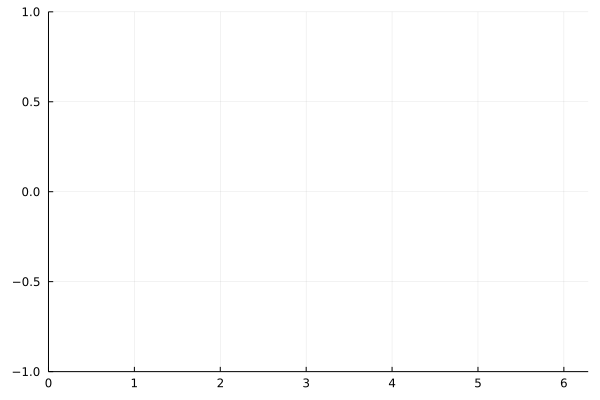# Functions, adding data, and animationsusing Plots
gr()

Plot multiple functions. You can also put the function first, or use the form plot(f, xmin, xmax) where f is a Function or AbstractVector{Function}.

Get series data: x, y = plt[i]. Set series data: plt[i] = (x,y). Add to the series with push!/append!.

Easily build animations. (convert or ffmpeg must be available to generate the animation.) Use command gif(anim, filename, fps=15) to save the animation.

p = plot([sin, cos], zeros(0), leg = false, xlims = (0, 2π), ylims = (-1, 1))
anim = Animation()
for x = range(0, stop = 2π, length = 20)
push!(p, x, Float64[sin(x), cos(x)])
frame(anim)
end

gif(anim, "assets/anim_gr_ex2.gif")